## Determinants of the PE ratio

In Chapter 17, the fundamentals that determine multiples were extracted using a discounted cash flow model – an equity model like the dividend discount model for equity multiples and a firm value model for firm multiples. The price earnings ratio, being an equity multiple, can be analyzed using an equity valuation model. In this section, the fundamentals that determine the price earnings ratio for a high growth firm are analyzed.

### A Discounted Cashflow Model perspective on PE ratios

In Chapter 17, we derived the PE ratio for a stable growth firm from the stable growth dividend discount model.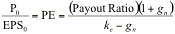If the PE ratio is stated in terms of expected earnings in the next time period, this can be simplified.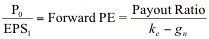The PE ratio is an increasing function of the payout ratio and the growth rate and a decreasing function of the riskiness of the firm. In fact, we can state the payout ratio as a function of the expected growth rate and return on equity.

Payout ratioSubstituting back into the equation above,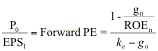The price-earnings ratio for a high growth firm can also be related to fundamentals. In the special case of the two-stage dividend discount model, this relationship can be made explicit fairly simply. When a firm is expected to be in high growth for the next n years and stable growth thereafter, the dividend discount model can be written as follows:where,

EPS0 = Earnings per share in year 0 (Current year)

g = Growth rate in the first n years

ke,hg = Cost of equity in high growth period

ke,st = Cost of equity in stable growth period

Payout = Payout ratio in the first n years

gn = Growth rate after n years forever (Stable growth rate)

Payout Ration = Payout ratio after n years for the stable firm

Divide both sides of the equation by EPS0.Here again, we can substitute in the fundamental equation for payout ratios.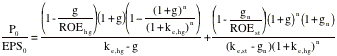where ROEhg is the return on equity in the high growth period and ROEst is the return on equity in stable growth:The left hand side of the equation is the price earnings ratio. It is determined by:

(a) Payout ratio (and return on equity) during the high growth period and in the stable period: The PE ratio increases as the payout ratio increases, for any given growth rate. An alternative way of stating the same proposition is that the PE ratio increases as the return on equity increases and decreases as the return on equity decreases.

(b) Riskiness (through the discount rate ke): The PE ratio becomes lower as riskiness increases.

(c) Expected growth rate in earnings, in both the high growth and stable phases: The PE increases as the growth rate increases, in either period.

This formula is general enough to be applied to any firm, even one that is not paying dividends right now. In fact, the ratio of FCFE to earnings can be substituted for the payout ratio for firms that pay significantly less in dividends than they can afford to.

###### Illustration 18.1: Estimating the PE ratio for a high growth firm in the two-stage model

Assume that you have been asked to estimate the PE ratio for a firm that has the following characteristics.

Growth rate in first five years = 25%               Payout ratio in first five years = 20%

Growth rate after five years = 8%                   Payout ratio after five years = 50%

Beta = 1.0                                                          Riskfree rate = T.Bond Rate = 6%

Required rate of return = 6% + 1(5.5%)= 11.5%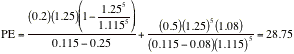The estimated PE ratio for this firm is 28.75. Note that the returns on equity implicit in these inputs can also be computed.

Return on equity in first 5 years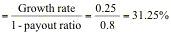Return on equity in stable growth###### Illustration 18.2: Estimating a Fundamental PE ratio for Procter and Gamble

The following is an estimation of the appropriate PE ratio for Procter and Gamble in May 2001. The assumptions on the growth period, growth rate and cost of equity are identical to those used in the discounted cash flow valuation of P&G in Chapter 13. The assumptions are summarized in Table 18.2.

Table 18.2: Summary Inputs for P& G

 High Growth Period Stable Growth Length 5 Forever after year 5 Cost of Equity 8.80% 9.40% Expected Growth Rate 13.58% 5.00% Payout Ratio 45.67% 66.67%

The current payout ratio of 45.67% is used for the entire high growth period. After year 5, the payout ratio is estimated based upon the expected growth rate of 5% and a return on equity of 15% (based upon industry averages).

Stable period payout ratio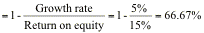The price-earnings ratio can be estimated based upon these inputs.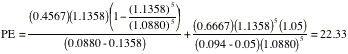Based upon its fundamentals, you would expect P&G to be trading at 22.33 times earnings. Multiplied by the current earnings per share, you get a value per share of \$66.99, which is identical to the value obtained in Chapter 13, using the dividend discount model.

#### PE Ratios and Expected Extraordinary Growth

The PE ratio of a high growth firm is a function of the expected extraordinary growth rate - the higher the expected growth, the higher the PE ratio for a firm. In Illustration 18.1, for instance, the PE ratio that was estimated to be 28.75, with a growth rate of 25%, will change as that expected growth rate changes. Figure 18.2 graphs the PE ratio as a function of the extraordinary growth rate during the high growth period.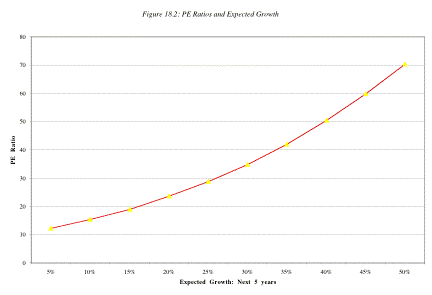As the firm's expected growth rate in the first five years declines from 25% to 5%, the PE ratio for the firm also decreases from 28.75 to just above 10.

The effect of changes in the expected growth rate varies depending upon the level of interest rates. In Figure 18.3, the PE ratios are estimated for different expected growth rates at four levels of riskless rates – 4%, 6%, 8% and 10%.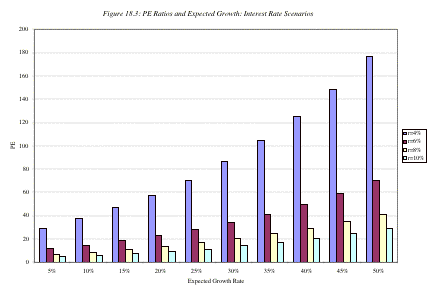The PE ratio is much more sensitive to changes in expected growth rates when interest rates are low than when they are high. The reason is simple. Growth produces cash flows in the future and the present value of these cash flows is much smaller at high interest rates. Consequently the effect of changes in the growth rate on the present value tend to be smaller.

There is a possible link between this finding and how markets react to earnings surprises from technology firms. When a firm reports earnings that are significantly higher than expected (a positive surprise) or lower than expected (a negative surprise), investors’ perceptions of the expected growth rate for this firm can change concurrently, leading to a price effect. You would expect to see much greater price reactions for a given earnings surprise, positive or negative, in a low-interest rate environment than you would in a high-interest rate environment.

#### PE ratios and Risk

The PE ratio is a function of the perceived risk of a firm and the effect shows up in the cost of equity. A firm with a higher cost of equity will trade at a lower multiple of earnings than a similar firm with a lower cost of equity.

Again, the effect of higher risk on PE ratios can be seen using the firm in Illustration 18.1. Recall that the firm, which has an expected growth rate of 25% for the next 5 years and 8% thereafter, has an estimated PE ratio of 28.75, if its beta is assumed to be 1.If you assume that the beta is 1.5, the cost of equity increases to 14.25%, leading to a PE ratio of 14.87:The higher cost of equity reduces the value created by expected growth.

In Figure 18.4, you can see the impact of changing the beta on the price earnings ratio for four high growth scenarios – 8%, 15%, 20% and 25% for the next 5 years.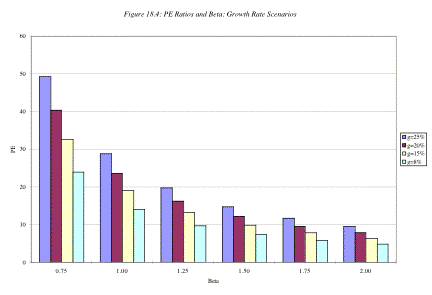As the beta increases, the PE ratio decreases in all four scenarios. However, the difference between the PE ratios across the four growth classes is lower when the beta is very high and increases as the beta decreases. This would suggest that at very high risk levels, a firm’s PE ratio is likely to increase more as the risk decreases than as growth increases. For many technology firms that are viewed as both very risky and having good growth potential, reducing risk may increase value much more than increasing expected growth.

 For purposes of simplicity, the beta and cost of equity are estimated to be the same in both the high growth and stable growth periods. They could have been different.